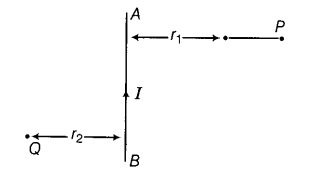# AB is a current-carrying conductor in the plane of the paper as shown in figure

AB is a current-carrying conductor in the plane of the paper as shown in figure. What are the directions of magnetic fields produced by it at points P and Q?Given r_{1}>r_{2}, where will the strength of the magnetic field be larger?

According to the right hand thumb rule, magnetic field at P is into the plane of paper and at Q it is out the plane of paper. The strength of the magnetic field at Q will be larger as strength of the
${\rm{field}};\alpha ;\frac{1}{{r({\rm{distance}})}}$